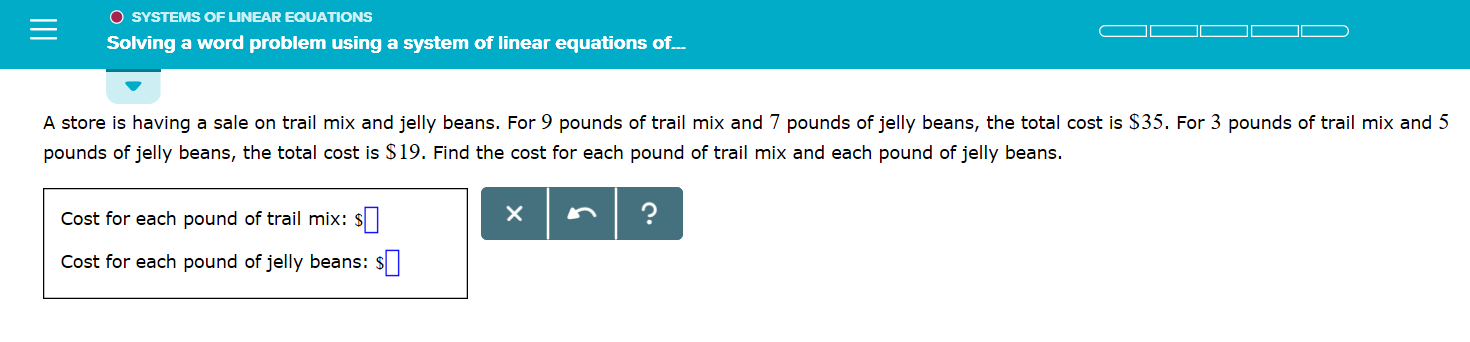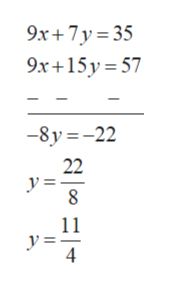O SYSTEMS OF LINEAR EQUATIONSSolving a word problem using a system of linear equations of.A store is having a sale on trail mix and jelly beans. For 9 pounds of trail mix and 7 pounds of jelly beans, the total cost is \$35. For 3 pounds of trail mix and 5pounds of jelly beans, the total cost is \$19. Find the cost for each pound of trail mix and each pound of jelly beans.Cost for each pound of trail mix::Cost for each pound of jelly beans:

Questionhelp_outlineImage TranscriptioncloseO SYSTEMS OF LINEAR EQUATIONS Solving a word problem using a system of linear equations of. A store is having a sale on trail mix and jelly beans. For 9 pounds of trail mix and 7 pounds of jelly beans, the total cost is \$35. For 3 pounds of trail mix and 5 pounds of jelly beans, the total cost is \$19. Find the cost for each pound of trail mix and each pound of jelly beans. Cost for each pound of trail mix:: Cost for each pound of jelly beans: fullscreen
Step 1

Let the cost for each pound of trail is \$x.

Let the cost for each pound of trail is \$y.

For 9 pounds of trail mix and 7 pounds of jelly beans, the total cost is \$35.

Step 2

For 3 pounds of trail mix and 5 pounds of jelly beans, the total cost is \$35.

Step 3

Multiply equation 2 multiply by 3 and...help_outlineImage Transcriptionclose9x+7y 35 9x+15y 57 -8y-22 22 y== 8 11 y==_ 4 fullscreen

Want to see the full answer?

See Solution

Want to see this answer and more?

Our solutions are written by experts, many with advanced degrees, and available 24/7

See Solution
Tagged in

Algebra